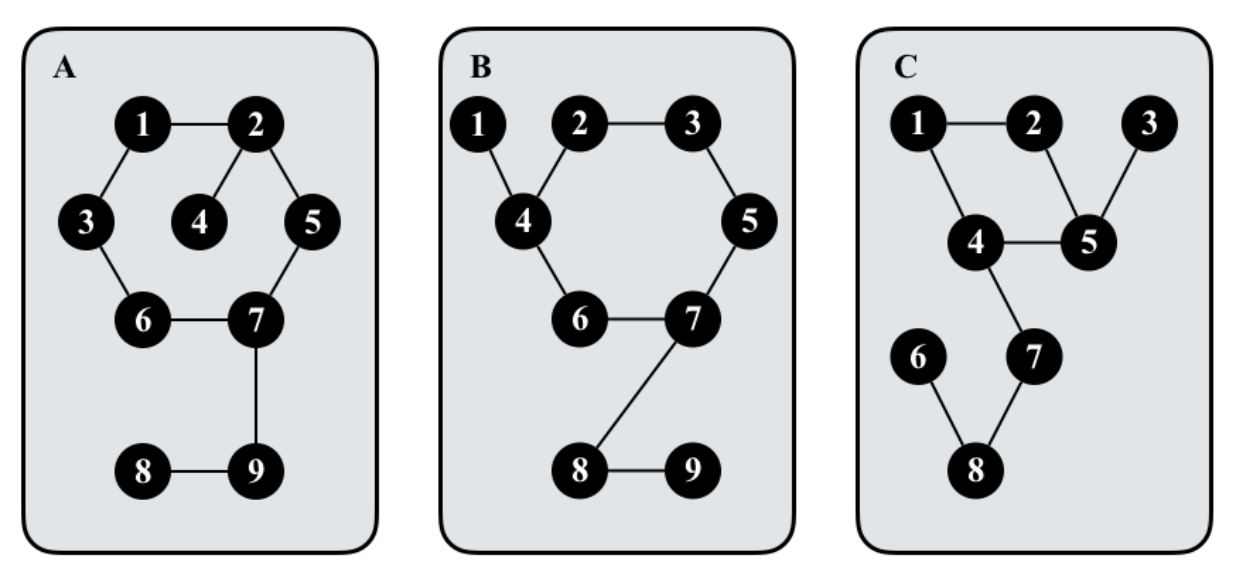시간 제한메모리 제한제출정답맞힌 사람정답 비율
30 초 1024 MB609423.529%

## 문제

A deck of graph cards is placed on the table. Each graph card χ is decorated with an undirected simple graph Gχ so that Gx is connected and Gχ has the same number of nodes and edges. Note that different graph cards may have different numbers of nodes. An example is depicted as follows.We say two graph cards are identical if and only if the graphs associated with them, say G1 = (V1, E1) and G2 = (V2, E2), are isomorphism; that is, there exists a bijection f between the node sets V1 and V2 so that for every x, y ∈ V1, edge (x, y) ∈ E1 if and only if edge (f(x), f(y)) ∈ E2. Our goal is to compute the number of distinct graph cards in the deck.

## 입력

The first line contains an integer t that indicates the number of test cases. For each test case, you are given a deck of graph cards. It begins with a line containing the number of graph cards n > 0. Then, n lines follow. Each line represents a graph card associated with a graph G in the following format:

k u1 v1 u2 v2 · · · uk vk

where k > 0 denotes the number of nodes (also edges) in G and for each i ∈ [1, k] (ui, vi) denotes an edge in G that connects nodes ui and vi. Note that the identifiers of nodes are integers in [1, k].

## 출력

For each test case, output the number of distinct graph cards in the given deck on a line.

## 제한

• 0 < t ≤ 30.
• 0 < n, k ≤ 106.
• For each test case, the numbers of nodes in the n graph cards sum up to at most 106.

## 예제 입력 1

1
2
4 1 2 2 3 3 1 1 4
4 1 2 2 3 3 1 2 4


## 예제 출력 1

1


## 예제 입력 2

2
2
4 1 2 2 3 3 1 1 4
5 1 2 2 3 3 1 2 4 2 5
3
9 1 2 2 5 5 7 7 6 6 3 3 1 2 4 7 9 9 8
9 1 4 4 2 2 3 3 5 5 7 7 6 6 4 7 8 8 9
9 1 2 2 5 5 4 4 1 4 7 7 8 8 6 8 9 5 3


## 예제 출력 2

2
2